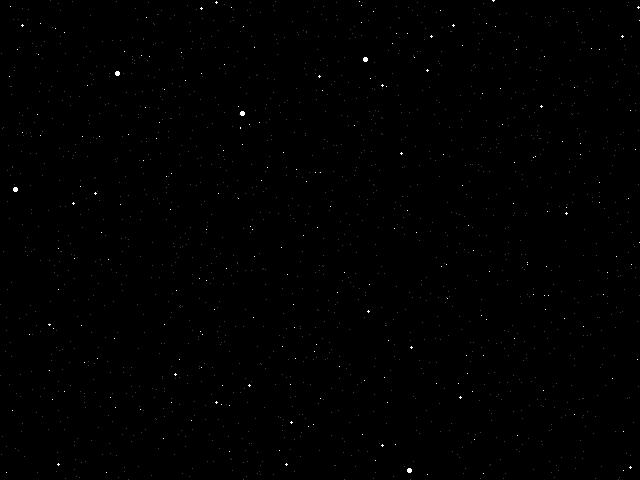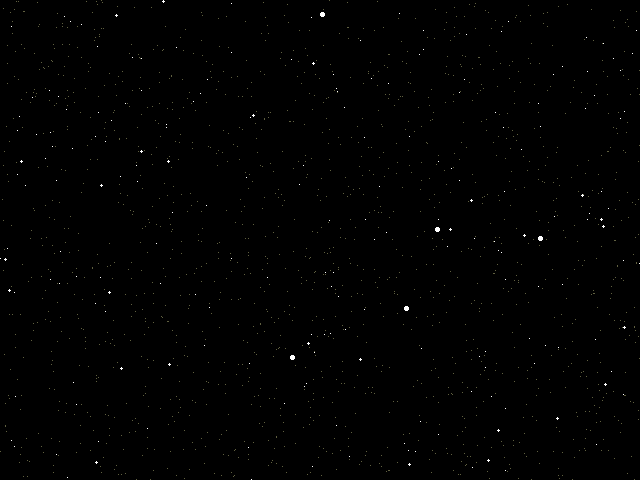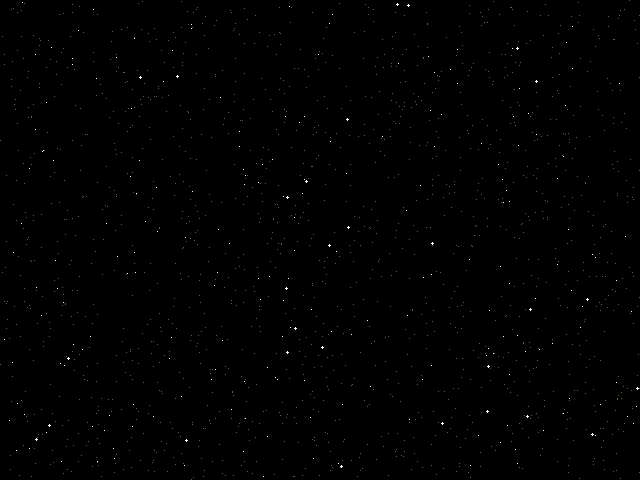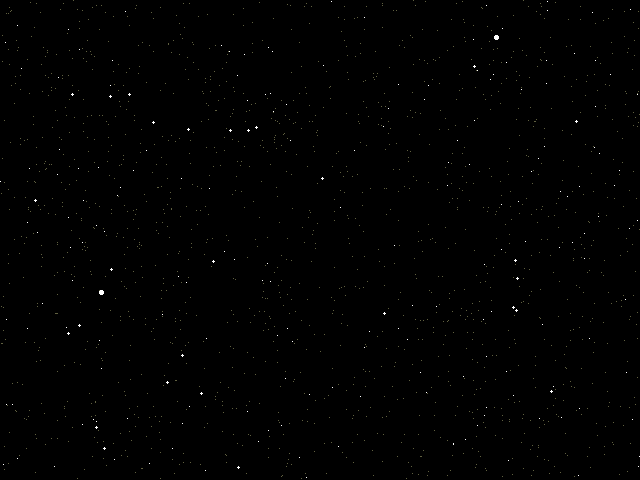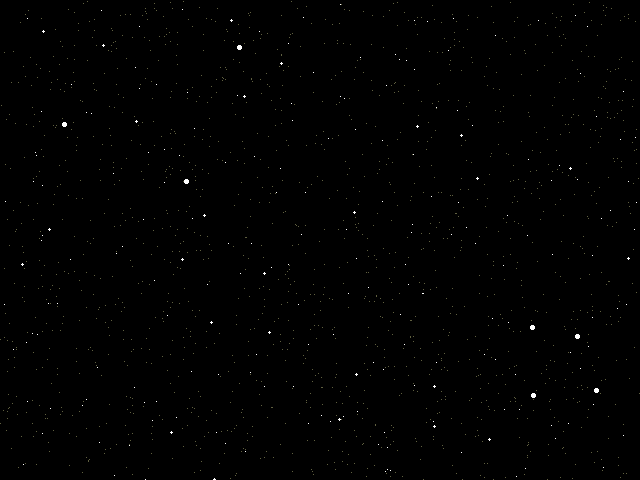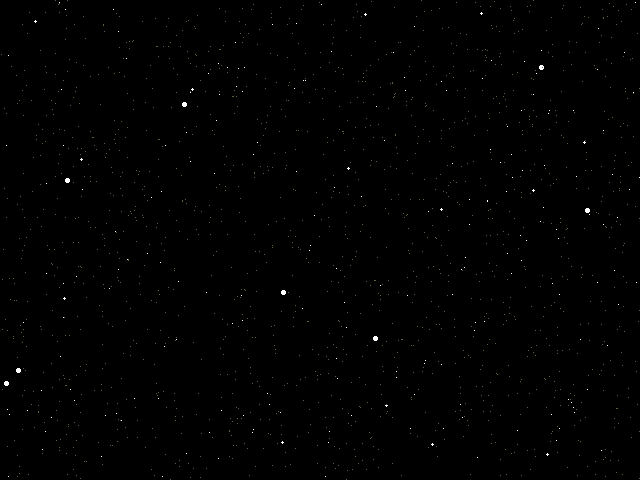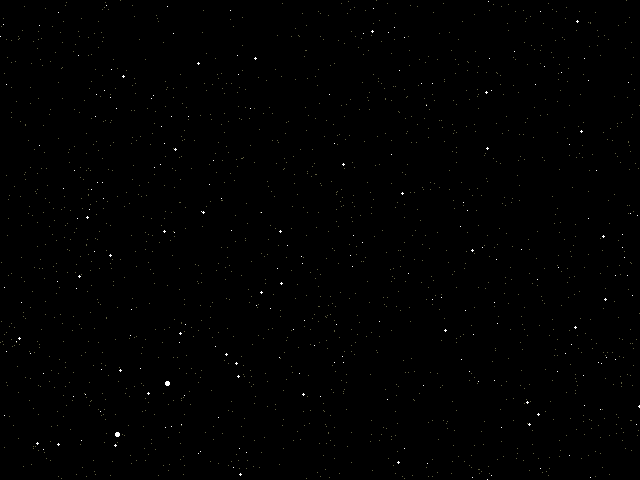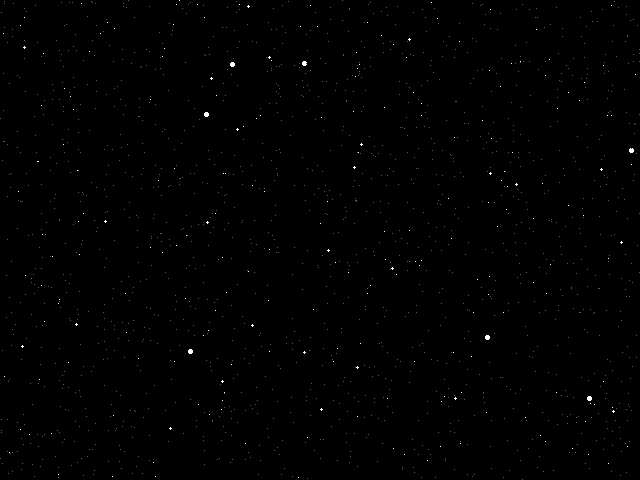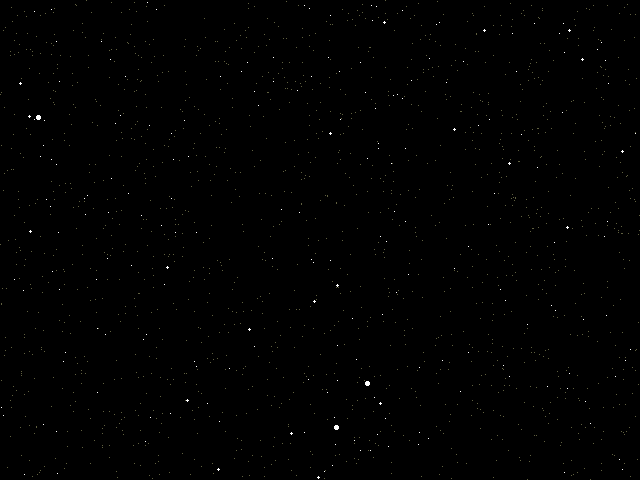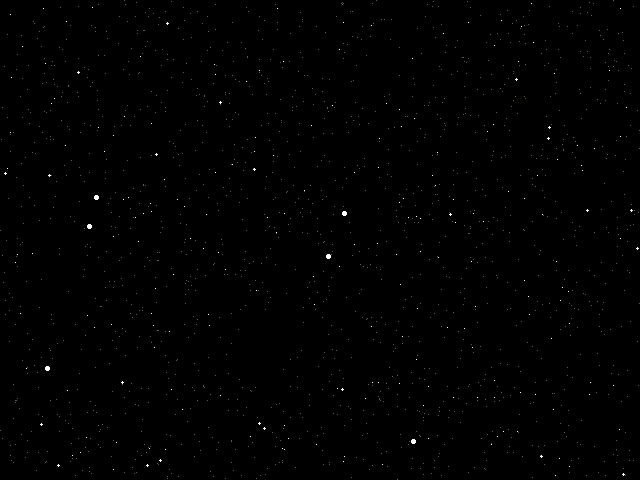### CYBER SKYDetails: Computer programs can be used to produce star images. A random number generator is used to determine both the position of a star in the "sky" and also its brightness. The apparent brightness of the star is varied by a combination of both the actual brightness of the point and its size. The sky images shown above and below were made with a very simple QBASIC program whose source code is given below so that you may create your own 'cyber sky images'. Study the program and note how the number of stars varies according to their brightness. ```'Generate starry sky [STARS.BAS] DIM level(3) 'magnitude levels RANDOMIZE TIMER 'so that each sky is different SCREEN 12 '640x480 pixels m1 = 3 'average number of mag 1 stars (default 3) r = 8 'star number ratio per magnitude (default 8) totalstars = m1 * (1 + r + r * r + r * r * r) '# of stars in the sky level(1) = m1 / totalstars 'compute levels to make level(2) = m1 * (1 + r) / totalstars '4 different magnitudes level(3) = m1 * (1 + r + r * r) / totalstars DO 'this is the loop that generates a new sky CLS 'clear screen/sky FOR i = 1 TO totalstars 'generate stars one by one xp = RND * 640 'x position on the screen yp = RND * 480 'y position on the screen magtst = RND 'generate a number to determine magnitude mag = 4 'start by assuming faintest star IF magtst < level(3) THEN mag = 3 'then brighten if IF magtst < level(2) THEN mag = 2 'generated random number is IF magtst < level(1) THEN mag = 1 'within specified level SELECT CASE mag 'now plot star according to magnitude CASE 4 PSET (xp, yp), 8 'faintest star (try changing colour to 7?) CASE 3 PSET (xp, yp), 15 'brightest star with a single point CASE 2 CIRCLE (xp, yp), 1, 15 'increase app brightness by increasing size PAINT (xp, yp), 15 CASE 1 CIRCLE (xp, yp), 1.5, 15 'brightest stars have largest area PAINT (xp, yp), 15 END SELECT NEXT i 'go back and plot all stars until finished DO a\$ = INKEY\$ 'wait for a keyboard input LOOP WHILE a\$ <> CHR\$(27) AND a\$ <> "q" 'ESC generates new sky LOOP WHILE a\$ <> "q" 'q quits program END ``` More computer generated star images are shown below. You might enjoy devising constellations for the star groupings.